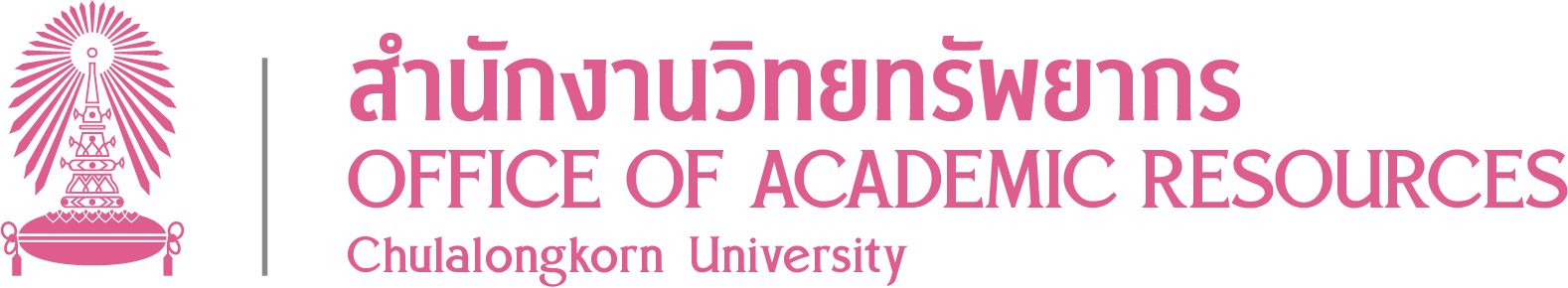Author Cohen, Y. Koby Problems in water distribution [electronic resource] : solved, explained, and applied / Y. Koby Cohen Boca Raton, Fla. : CRC Press, c2002 http://marc.crcnetbase.com/isbn/9781420056563 240 p. : ill

CONTENT

Machine generated contents note: Chapter 1 Basic Math for the Distribution System Operator Significant Figures Procedures for Determining the Number of Significant Figures Significant Figures Practice Problems Rounding Numbers Procedures for Rounding Numbers Rounding Numbers Practice Problems Addition, Subtraction, Multiplication, and Division Addition Practice Problems Subtraction Practice Problems Multiplication Practice Problems Division Practice Problems Basic Distribution System Problems and Solutions Type A - Unit Conversion Problems Procedures for Solving Unit Conversion Problems Type B - Quantity Problems Procedures for Solving Quantity Problems Type C - Percent Problems Procedures for Solving Percent Problems Type D - Arithmetic Average Problems Procedures for Solving Arithmetic Average Problems Chapter 2 Advanced Math for the Distribution System Operator Type E - Pressure and Elevation Head Problems Procedures for Solving Pressure/Elevation Head Problems Type F - Velocity and Flow Problems Procedures for Solving Velocity/Flow Problems Type G - Blending/Mixing Problems Procedures for Solving Blending/Mixing Problems Type H - Chemical Amount and Application Rate Problems Procedures for Solving Chemical Amount and Application Rate Problems Chapter 3 Unit Conversion Problems Unit Conversion Problems and Solutions Unit Conversion - Practice Exam Chapter 4 Distribution Math Problems - Part 1 Type B, C, and D Problems and Solutions Distribution Math Problems, Part 1 - Practice Exam Chapter 5 Distribution Math Problems - Pt 2 Type E through H Problems and Soluins Distribution Math Problems, Part 2 - Practice Ex Chapter 6 Distribution Knowledge Problems Distribution Knowledge Problems and Solutions Distribution Knowledge Problems - Practice Exam Chapter 7 Water Quality Knowledge Problems Water Quality Knowledge Problems and Solutions Water Quality Knowledge - Practice Exam Chapter 8 Federal Regulations Federal Regulations Problems and Solutions Federal Regulations - Practice Exam Chapter 9 Safety Knowledge Problems Safety Knowledge Problems and Solutions Safety Knowledge - Practice Test Chapter 10 Operation and Maintenance Problems Operation and Maintenance Problems and Solutions Operation and Maintenance - Practice Test Appendix A Abbreviations Metric Symbols and Multiplication Factors Appendix B Conversions Length Conversions Area Conversions Volume Conversions Flow Conversions Weight Conversions Pressure Conversions Appendix C Formulas Area Formulas Volume Formulas Appendix D References AWWA Manuals AWWA Standards Monographs Appendix E Water Industry Organizations Appendix F State Drinking Water Programs Appendix G Common Chemicals Used for Water Treatment Appendix H Solutions to Problems Index

Water -- Distribution -- Problems exercises etc Water quality management -- Problems exercises etc Electronic books.